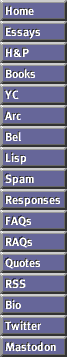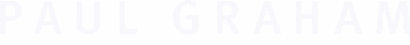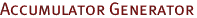Revenge of the Nerds yielded a collection of canonical solutions to the same problem in a number of languages.The problem: Write a function foo that takes a number n and returns a function that takes a number i, and returns n incremented by i.Note: (a) that's number, not integer, (b) that's incremented by, not plus. C++ template struct Acc { Acc(T n) : n(n) {} template Acc(const Acc& u) : n(u.n) {} template T operator()(U i) { return n += i; } T n; }; template Acc foo(T n) { return Acc(n); } Dylan define function foo (n) method (i) n := n + i end; end function; E def foo (var n) :any { def inc (i) :any { n += i } } Erlang foop(N)-> receive {P,I}-> S =N+I, P!S, foop(S) end. foo(N)-> P=spawn(foo,foop,[N]), fun(I)-> P!{self(),I}, receive V->V end end. Haskell import IOExts foo n = do r <- newIORef n return (\i -> do modifyIORef r (+i) readIORef r) Javascript function foo (n) { return function (i) { return n += i } } Lisp: Arc (def foo (n) [++ n _]) Lisp: Common Lisp (defun foo (n) (lambda (i) (incf n i))) Lisp: Goo (df foo (n) (op incf n _)) Lisp: Scheme (define (foo n) (lambda (i) (set! n (+ n i)) n)) Lua function foo(n) return function (i) n = n + i return n end end Maple foo := proc(n) local s; s := n; proc(i) s := s + i end end Mathematica foo = Module[{s=#},s+=# &] & Mozart fun {Foo N} A = {NewCell N} in fun {\$ B} C D in {Exchange A C D} if {IsInt C} andthen {IsFloat B} then D = {IntToFloat C}+B elseif {IsFloat C} andthen {IsInt B} then D = C+{IntToFloat B} else D = C+B end {Access A} end end NewtonScript foo := func (n) func (i) n := n + i ; Perl 5 sub foo { my (\$n) = @_; sub {\$n += shift} } Python class foo: def __init__(self, n): self.n = n def __call__(self, i): self.n += i return self.n Rebol foo: func [ n ] [ func [ i ] [ n: n + i ] ] Ruby def foo (n) lambda {|i| n += i } end Smalltalk foo: n |s| s := n. ^[:i| s := s + i. ] VBScript Class acc Private n Public Default Function inc(i) n = n + i inc = n End Function End Class Function foo(n) Dim bar Set bar = New acc bar(n) Set foo = bar End Function Some languages are not represented here, either because you can't write this program in them (short of Greenspun's Tenth Rule) or because no one has yet sent me the code for that language. Please don't send may any new submissions for the time being; I don't have time to look at them. Credits: C++, Brendan Corfman and Daniel Cowgill; Dylan, Neel Krishnaswami; E, Darius Bacon; Erlang, Heinz Eriksson; Goo, Jonathan Bachrach; Haskell, Malcolm Wallace and Tom Pledger; Javascript, Anton van Straaten; Lua, Chris Laurel; Maple, Stefan Vorkoetter; Mathematica, Kovas Boguta; Mozart, Kari Pahula; NewtonScript, Sean Luke; Perl, Dan Giffin and Trevor Blackwell; Python, Jeremy Hylton; Rebol, Andreas Bolka; Ruby, Stephan Schmidt; Smalltalk, Trevor Blackwell; VBScript, Stefan Holm.